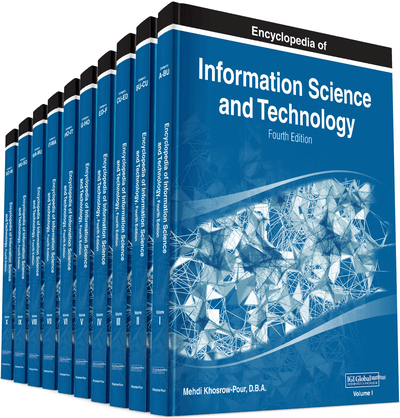# Decimal Hardware Multiplier

Mário Pereira Vestias (Instituto Politécnico de Lisboa, Portugal)
Copyright: © 2018 |Pages: 12
DOI: 10.4018/978-1-5225-2255-3.ch400
OnDemand PDF Download:
Available
\$37.50
No Current Special Offers

## Abstract

IEEE-754 2008 has extended the standard with decimal floating point arithmetic. Human-centric applications, like financial and commercial, depend on decimal arithmetic since the results must match exactly those obtained by human calculations without being subject to errors caused by decimal to binary conversions. Decimal Multiplication is a fundamental operation utilized in many algorithms and it is referred in the standard IEEE-754 2008. Decimal multiplication has an inherent difficulty associated with the representation of decimal numbers using a binary number system. Both bit and digit carries, as well as invalid results, must be considered in decimal multiplication in order to produce the correct result. This article focuses on algorithms for hardware implementation of decimal multiplication. Both decimal fixed-point and floating-point multiplication are described, including iterative and parallel solutions.
Chapter Preview
Top

## Background

Usually, humans perform arithmetic operations using decimal arithmetic. However, computers do it with binary arithmetic. It means that performing decimal operations in a computer without support for decimal arithmetic is subject to errors from representing decimal numbers, converting them and rounding. In fact, it is easy find decimal numbers that cannot be represented exactly in binary format (e.g., 0.1). Several examples exist where errors due to binary calculation of decimal numbers are obtained. A clarifying example came from the Vancouver Stock Exchange (Quinn, K., 1983), where due to rounding errors an initial index value of 1000.000 dropped to 574.081 instead of the correct result of 1098.892.

In fact, the business and commercial markets were one of the triggers for the importance of decimal computer arithmetic since many commercial databases have more than 50% of the numerical data represented in decimal (Tsang, A. & Olschanowsky, M., 1991). In these cases, to avoid errors with undesirable consequences it is important to have a complete system to support decimal arithmetic.

At the era of electronic computers, both binary and decimal arithmetic functions were considered. We had computer systems, like the ENIAC (Goldstine, H. & Goldstine, A., 1996) and IBM 650 (Knuth, D., 1986) implementing arithmetic functions in decimal, and others like EDSAC (Wilkes, M., 1997) and EDVAC (Williams, M., 1993) that adopted binary based arithmetic implementations. Both arithmetic systems were still considered after the advent of transistorized computers with decimal numbers represented with four bits following different representations, like in Binary-Coded Decimal (BCD) format. However, soon binary arithmetic was adopted by most computer systems since at that time scientific computing, whose operations could be more efficiently implemented in binary, were more in demand than financial computing that requires decimal arithmetic to avoid costs from representation errors. So, binary became very popular, while decimal was supported only by some computers in the 1960s and 1970s.

Precise decimal arithmetic operations with binary based computing systems are done in software. In some cases, these binary-based computing systems include some specific hardware instructions that are hardware supported and so software algorithms can take advantage of them to speed-up execution. Several languages include primate decimal datatypes, including Ada, COBOL, and SQL. Several other languages support the GDAS (General Decimal Arithmetic Specification) (Cowlishaw, M., 2008), including the IBM C DecNumber Library (Cowlishaw), the Java BigDecimal (Sun Microsystems), Eiffel Decimal Arithmetic (Crismer), Python Decimal (Batista), among others. Decimal floating point extensions conforming to the IEEE 754-2008 standard were proposed for C (JTC 1, 2007) and C++ (JTC 1, 2008) languages. These extensions were supported by GNU C compiler 4.2 release. Intel has also developed a decimal floating-point math library (Intel) that implements decimal floating-point arithmetic specified in IEEE 754-2008.

## Key Terms in this Chapter

Iterative Decimal Multiplication: A decimal multiplication method where partial products are generated and accumulated step-by-step in an iterative process.

Decimal Multiplication: Refers to the multiplication operation where the operands are represented with some decimal digit encoding.

Parallel Decimal Multiplication: A decimal multiplication method where partial products are generated and added according to a parallel process.

Densely Packed Decimal: An encoding scheme in which three BCD digits are represented with ten bits.

Decimal Fixed-Point Multiplication: Refers to the multiplication operation where the inputs are represented in radix ten fixed-point numbers.

Decimal Floating-Point Multiplication: Refers to the multiplication operation where the inputs are represented in radix ten real numbers.

Binary-Coded Decimal: A binary code of decimal numbers where each digit is represented with four bits.

## Complete Chapter List

Search this Book:
Reset